Courses

# Test: Soil Mechanics- 1

## 10 Questions MCQ Test GATE Civil Engineering (CE) 2022 Mock Test Series | Test: Soil Mechanics- 1

Description
This mock test of Test: Soil Mechanics- 1 for Civil Engineering (CE) helps you for every Civil Engineering (CE) entrance exam. This contains 10 Multiple Choice Questions for Civil Engineering (CE) Test: Soil Mechanics- 1 (mcq) to study with solutions a complete question bank. The solved questions answers in this Test: Soil Mechanics- 1 quiz give you a good mix of easy questions and tough questions. Civil Engineering (CE) students definitely take this Test: Soil Mechanics- 1 exercise for a better result in the exam. You can find other Test: Soil Mechanics- 1 extra questions, long questions & short questions for Civil Engineering (CE) on EduRev as well by searching above.
QUESTION: 1

### If the plasticity index of a soil is 45%, then the soil will be:

Solution: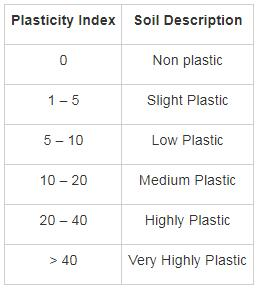QUESTION: 2

### Consider the following statements. The incorrect statement is:

Solution:

Water content is the ratio of the mass of water to the mass of solids.

It can be greater than 100%.

The degree of saturation has ranged from 0% to 100%.

The porosity cannot be greater than 100%.

The void ratio of soil can be greater than 1.

QUESTION: 3

### The term ‘Loess’ indicates those soils which are 1.  Uniformly graded 2.  Poorly graded 3.  Made up of more than 50% sand size particles 4. Made up of more than 50% of silt particles 5. These are transported by winds Which of the above statements are correct:

Solution:

Loess is an Aeolian Sediment i.e. transported by winds.

These contain fine particles like silt and clay (typically in the 20–50 micrometer size range).

It is usually homogeneous and highly porous and is traversed by vertical capillaries that permit the sediment to fracture and form vertical bluffs.

QUESTION: 4

Match List – I (Type of soil) with List-II (Mode of transportation and deposition) and select the correct answer using the codes given below the lists: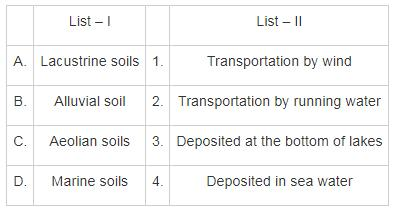Solution:

Lacustrine soil: Soil which is deposited from suspension in fresh still water of the lakes is known as Lacustrine soil.

Alluvial Soil: Soil which is been deposited from suspension in running water is known as Alluvial soil. It is transported soil formed by physical wheathering.

Aeoline Soil: Soil which is formed due to transportation by wind is known as Aoeline soil. It is also a transported soil.

Marine soil: Soil which is deposited from the suspension in sea water is known as marine soil.

QUESTION: 5

In wet soil mass, air occupies one-sixth of its volume and water occupies one – third of its volume. The void ratio of the soil is

Solution:

Void ratio, e = Vv/Vs

Vv = air void + water filled voids
Calculation: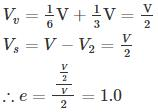QUESTION: 6

The given figure indicate the weights of different pycnometers: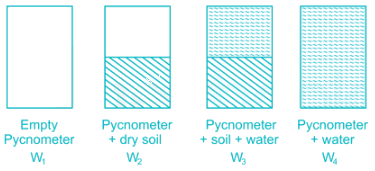The specific gravity of the solids is given by

Solution:

Specific gravity of solids is the ratio of weight of given volume of solids to the weight of equivalent volume of water at 4° C.

Specific gravity is usually reported at 27°C and determined using pycnometer. It is given by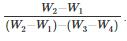*Answer can only contain numeric values
QUESTION: 7

The following data are given for soil:

• Porosity: n = 0.4

• The specific gravity of the soil solids: Gs = 2.68

• Moisture content: w = 12%

Determine the mass of water in kg to be added to 10 m3 of soil for full saturation.

Solution: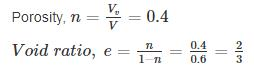Total volume of soil, V = 10 m3

∴ VV = 0.4 × 10 = 4m3

Volume of solids, Vs = V – VV = 10 – 4 = 6 m3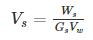Mass of solids, Ws = Vs Gs Yw = 6 × 2.68 × 1000 = 16080 kg

Moisture content = 0.12

So, Mass of water = 0.12 × 16080 = 1929.6 kg

Volume of water = 1929.6/1000 = 1.929 m3

So, phase diagram can be shown as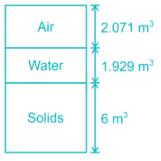For full saturation, the amount of air has to be replaced by water.

So, water to be added = 2.071 × 1000 = 2071 kg

QUESTION: 8

The water content of a soil sample is found to be 35%. If the percentage of air voids present in the soil sample is 5%. Then compute dry unit weight and air content of the soil sample when the void ratio of the soil sample is 0.65.

Take specific gravity of soil solids and unit weight of water to be 2.70 and 10 kN/m3.

Solution:

Dry unit weight is given by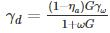ηa = 0.05

G = 2.70

ω = 0.35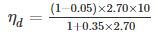ηd = 13.19 kN/m3

ηa = acη

η → porosity

ac → aircontent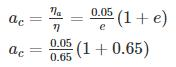ac = 0.1269
ac = 12.70%

*Answer can only contain numeric values
QUESTION: 9

A fully saturated clay has a water content of 40% and unit weight 19 kN/m3. After oven drying the dry density of the soil becomes 18 kN/m3. The shrinkage limit for the soil sample is ______%. (Take γw=γw=10 kN/m3)

Solution: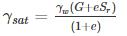And, e sr = wG

⇒ e × 1 = 0.40 G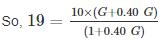⇒ G = 2.97
Shrinkage limit, w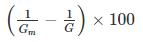Where, Gm = Mass specific gravity at dry state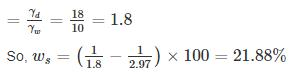*Answer can only contain numeric values
QUESTION: 10

The soil has to be excavated from a borrow-pit which has a density of 1.12 gm/cc with a water content of 12% to fill a land. The soil is compacted to a density of 1.32 gm/cc with water content 20%. Find the volume of soil to be excavated in m3 for 1000 m3 volume of the fill?

Solution: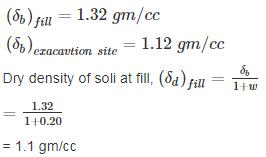Dry density of soil at excavation site,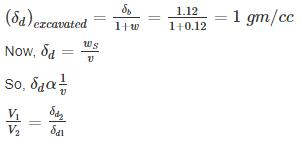∴ Volume of excavated soil =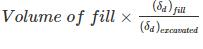= 1000 × 1.1/1
= 1100 m3